# Atomic Structure and Periodic TrendsPage 3

#### WATCH ALL SLIDES

and show properties such as interference,

diffraction

Slide 20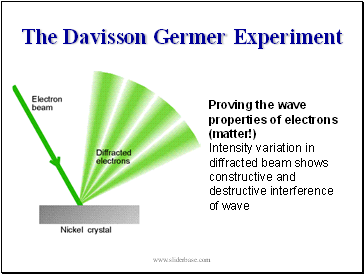The Davisson Germer Experiment

Proving the wave properties of electrons (matter!)

Intensity variation in diffracted beam shows constructive and destructive interference of wave

Slide 21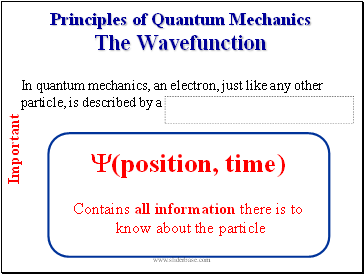Principles of Quantum Mechanics The Wavefunction

Y(position, time)

In quantum mechanics, an electron, just like any other particle, is described by a

Contains all information there is to

Important

Slide 22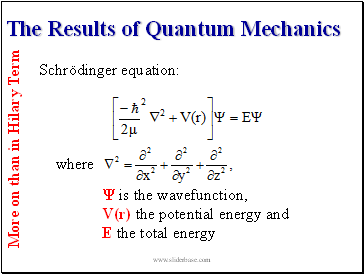## The Results of Quantum Mechanics

 is the wavefunction,

V(r) the potential energy and

E the total energy

More on than in Hilary Term

Slide 23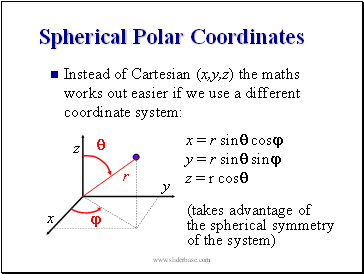## Spherical Polar Coordinates

Instead of Cartesian (x,y,z) the maths works out easier if we use a different coordinate system:

y

x

z

x = r sin cos

y = r sin sin

z = r cos

the spherical symmetry

of the system)

Slide 24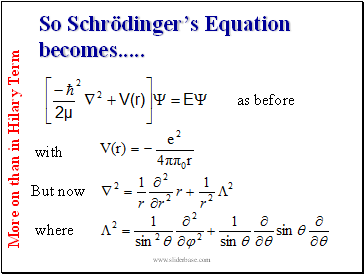So Schrödinger’s Equation becomes .

More on than in Hilary Term

Slide 25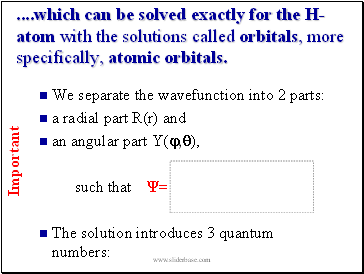We separate the wavefunction into 2 parts:

an angular part Y(,),

such that =

The solution introduces 3 quantum numbers:

Important

which can be solved exactly for the H-atom with the solutions called orbitals, more specifically, atomic orbitals.

Slide 26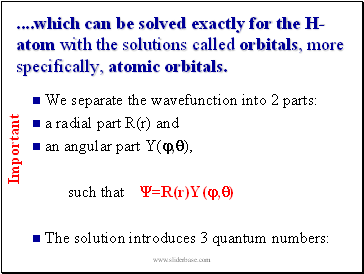which can be solved exactly for the H-atom with the solutions called orbitals, more specifically, atomic orbitals.

We separate the wavefunction into 2 parts:

an angular part Y(,),

such that =R(r)Y(,)

The solution introduces 3 quantum numbers:

Important

Slide 27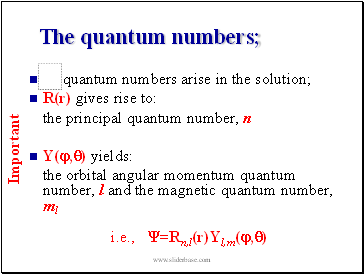## The quantum numbers;

quantum numbers arise in the solution;

R(r) gives rise to:

the principal quantum number, n

Y(,) yields:

the orbital angular momentum quantum number, l and the magnetic quantum number, ml

i.e., =Rn,l(r)Yl,m(,)

Important

Slide 28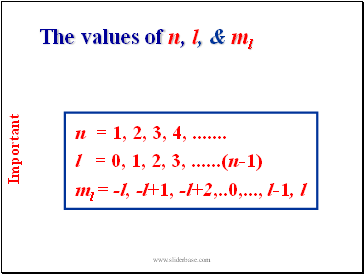The values of n, l, & ml

Go to page:
1  2  3  4  5  6  7  8  9  10  11  12  13  14  15
16  17